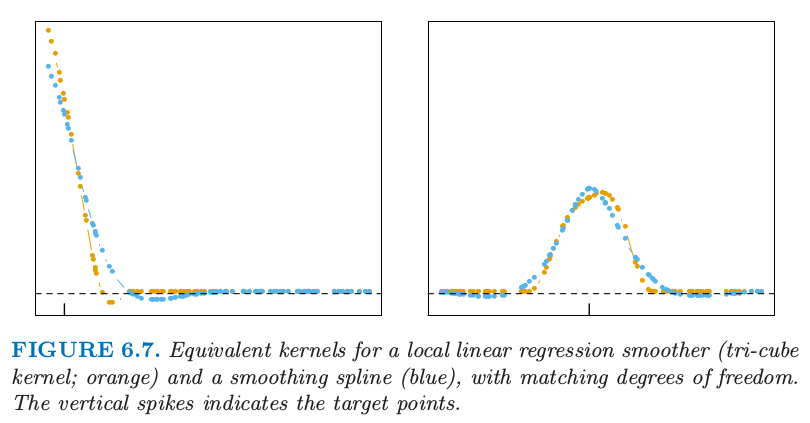# 6.2 选择核的宽度¶

• 对于有度量宽度的 Epanechnikov 或三次立方核，$\lambda$ 为支撑域的半径．
• 对于高斯核，$\lambda$ 为标准差．
• $\lambda$ 为 $k$-最近邻中最近邻的数目 $k$，经常表达成分数或者整个训练样本的跨距 $k/N$．

• 如果窗口较窄，$\hat f(x_0)$ 是离 $x_0$ 近的一小部分 $y_i$ 值的平均，则它的方差相对较大——与单个 $y_i$ 的方差相近．偏差会趋向于较小，这是因为 $\E(y_i)=f(x_i)$ 中的每一个应该与 $f(x_0)$ 相近．
• 如果窗口较宽，$\hat f(x_0)$ 的方差相对于任意 $y_i$ 的方差较小，这是因为平均的效应．偏差会较高，因为我们现在采用的是与 $x_0$ 离得较远的观测 $x_i$，而且不保证 $f(x_i)$ 离 $f(x_0)$ 较近．

weiya 注：Ex. 6.7